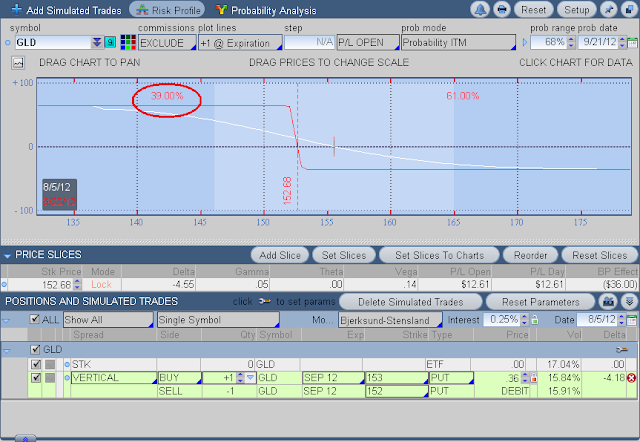## Sunday, August 5, 2012

### finding the vig in vertical spreads

vigorish (or vig in gambling parlance) is the house cut on a bet pay-out. if there is a theoretical even-money payout on a bet then, by decree, the house always pays a little less. this way the house creates a sustainable business without charging fees, because their risk/reward ratio is engineered to be less than their win/loss ratio (more on this below.) now the fact that brokerages do charge a fee for play, i.e. the commission, maybe implies that their vig is not so certain or as big as the casino's. to be able to judge what a brokerage's vig is at all you would need a way to determine even-moneyness, which when it comes to pure stock pricing is as subjective as it gets. however, for certain kinds of option trades, namely vertical spreads, it is possible to calculate a theoretical even-money price using the thinkdesktop analyze tab. here's how:

bring your perspective vertical spread into the analyze tab by setting up a trade in the trade tab and then select analyze duplicate trade in the drop down you get from clicking the button just to the left of the trade on the trade tab (make sure the price lock is in the unlocked position so you get the mark, or mid-bid/ask, price for the spread.)gld credit vertical in trade tab
now, in the analyze tab you will need to set up two components - the probability date is adjusted to expiration friday, which, in this case, is sept. 21, and, the slices are set to break-even on sept. 22 (the saturday after friday expiration.)gld credit spread in analyze tab
the even money price of this vertical spread can be determined directly from the probabilities. since this is a credit spread I take the probability of loss and multiply by the width of the strikes thus: .39 x \$1.00 = .39 (if this had been a \$2 wide spread then the even-money price would have been .78.) so, if even money on this spread is 39 cents and it is selling for 36 cents i discern that there is a 3 cent vig for the buy-side of this spread. that buyer may or may not be the market maker, i don't know. what this does tell me is that the credit i am receiving is very close to the worth of the risk i am taking, if i have to hold into expiration. since the probability of a win is 61% then the laws of chance favor a run of winning luck on this side of the trade, thus, the vig is maybe worth-the-while.

now, for a debit spread the calculation is slightly different. i take the probability of winning and multiply by width of the strikes thus: .39 * 1 = .39 as shown below for the buy-side of this same spread:gld debit vertical in analyze tab.
this says that the price i am paying for the potential reward is priced at a 3 cent discount to the even money chances of winning on this spread, if held to expiration. i will have to sit for a 61% chance of a loss on this trade which laws of chance would favor for runs of losing trades on repeated undertakings of this type of trade, but on large numbers of such trades I should come out 3 cents ahead, excluding commissions.

geeky mathematical aside:

an even-money trade occurs when the win/loss ratio equates to the risk/reward ratio or:
(1)   win/loss = risk/reward
if i am trading even-money then there is no free lunch. if i win frequently then i should lose big on the occasional loss OR if i lose frequently then i should win big on the occasional win.
(2) risk = strikewidth - reward
this is the definition of a the vertical spread. the expiration price of the spread can be no greater than the strike-price differential between the short and long option. thus reward+risk must equate to strike price width.
(3) win probability = 1 - losing probability
something's gotta happen, a win or a loss. i discount the miniscule chance that the price of the underlying is exactly the break-even price at expiration - that's a little like the chances of a flipped coin landing on the edge.

now, by substituting (2) and (3) into (1), i get:
(4) (1 - loss)/loss = (strikewidth-reward)/reward
now, by dividing each term of the parenthetical expressions in (4) by the respective divisors, i get:
(5)  1/loss -1 = strikewidth/reward -1
finally, by adding 1 to both sides of (5) and solving for reward, i get:
(6) reward = strikewidth*loss probability
this is the math of the method of finding the even-money price of a credit spread that i demonstrated above.

if your eyes haven't glazed over from the sheer geekiness of the above, relatively simple, high school algebra, then, you might find it a worthy exercise to show that:
(7) risk = strikewidth*win probability

the even money price of a debit vertical.